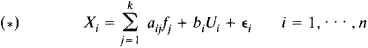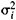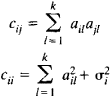# factor analysis

Also found in: Dictionary, Thesaurus, Medical, Legal, Financial, Acronyms, Wikipedia.

## factor analysis

[′fak·tər ə‚nal·ə·səs]
(mathematics)
Given sets of variables which are related linearly, factor analysis studies techniques of approximating each set relative to the others; usually the variables denote numbers.
McGraw-Hill Dictionary of Scientific & Technical Terms, 6E, Copyright © 2003 by The McGraw-Hill Companies, Inc.

## factor analysis

a MULTIVARIATE statistical technique in which the covariances (or CORRELATIONS) between a large set of observed VARIABLES are explained in terms of a small number of new variables called factors. The ideas originated in the work on correlation by Galton and Spearman, and were developed primarily in studies of intelligence. Most applications are found in psychology and sociology.

The technique is ‘variable directed’, with no distinction between INDEPENDENT and DEPENDENT VARIABLES in the data set. There are four steps to the analysis. The first is to derive a correlation matrix in which each variable in the data set is correlated with all the other variables. The next step is to extract the factors. The aim of this stage is to determine the minimum number of factors that can account adequately for the observed correlations between the original variables. If the number of factors identified is close to the number of original variables, there is little point to the factor analysis. Sometimes it is difficult to assign a meaningful name to the factors. The purpose of the third (optional) step, rotation, is to find simpler and more easily interpretable factors. If a satisfactory model has been derived, the fourth step is to compute scores for each factor for each case in the data set. The factor scores can then be used in subsequent analyses.

Factor analysis attracts a lot of criticism (Chatfield and Collins, 1980). The observed correlation matrix is generally assumed to have been constructed using product moment correlations. Hence, the usual assumptions of an interval measurement, normal distributions and homogeneity of variance are needed. Against this, it is argued the technique is fairly robust. Another problem is that the different methods of extraction and rotation tend to produce different solutions. Further, although factors may be clearly identified from the analysis, it may be difficult to give them a meaningful interpretation. Despite the need for so many judgmental decisions in its use, factor analysis remains a useful exploratory tool.

Collins Dictionary of Sociology, 3rd ed. © HarperCollins Publishers 2000
The following article is from The Great Soviet Encyclopedia (1979). It might be outdated or ideologically biased.

## Factor Analysis

a branch of multivariate analysis embracing methods for estimating the dimensions of a set of observed variables by studying the structure of the covariance or correlation matrices.

The basic assumption underlying factor analysis is that the correlations between a large number of observable variables are determined by the existence of a smaller number of hypothetical unobservable variables, or factors. A general model for factor analysis is provided in terms of the random variables X1 . . .,Xn, which are the observation results, by the following linear model:Here, the random variables fj are common factors, the random variables Ui are factors specific to the variables Xi and are not correlated with the fj, and the εj, are random errors. It is assumed that k < n, that the random variables ej are independent of each other and of the fj and Ui, and that E∊i = 0 and D∊i =The constant coefficients aij are called loadings (weights): aij is the loading of the ith variable on the jth factor. The quantities aij, bi, andare taken as unknown parameters that have to be estimated.

In the form given above, the model for factor analysis is characterized by some indeterminacy, since n variables are expressed in terms of n + k other variables. Equations (*), however, imply a hypothesis, regarding the covariance matrix, that can be tested. For example, if the factors fj are uncorrelated, Dfi = 1, Bi = 0, and cij are the elements of the matrix of covariances between the Xi, then there follows from equation (*) an expression for the cij in terms of the loadings and the variances of the errors:The general model for factor analysis is thus equivalent to a hypothesis regarding the covariance matrix: the covariance matrix can be represented as the sum of the matrix A A’ and the diagonal matrix with elements, where

A = {aij}

The estimation procedure in factor analysis consists of two steps. First, the factor structure (that is, the number of factors required to account for the correlations between the Xi) is determined, and the loadings are estimated. Second, the factors are estimated on the basis of the observation results. The fundamental obstacle to the interpretation of the set of factors is that for k > 1 neither the loadings nor the factors can be determined uniquely, since the factors fj in equations (*) can be replaced by means of any orthogonal transformation. This property of the model is made use of to transform (rotate) the factors; the transformation is chosen so that the observed variables have the maximum possible loadings on one factor and minimum possible loadings on the remaining factors.

Various practical methods are known for estimating loadings. The methods assume that X1,. . ., Xn obey a multivariate normal distribution with covariance matrix C = {cij}. The maximum likelihood method is noteworthy. It leads to a unique set of estimates of the cij, but for the estimates of the aij it yields equations that are satisfied by an infinite set of solutions with equally good statistical properties.

Factor analysis is regarded as dating from 1904. Although it was originally developed for problems in psychology, the range of its applications is much broader, and it is now used to solve various practical problems in such fields as medicine, economics, and chemistry. A rigorous theoretical grounding, however, has not yet been provided for many results and methods of factor analysis that are widely used in practice. The mathematical description of modern factor analysis in a rigorous manner is an extremely difficult task and remains uncompleted.

### REFERENCES

Lawley, D., and A. Maxwell. Faktornyi analiz kak statisticheskii metod. Moscow, 1967. (Translated from English.)
Harman, H. Sovremennyi faktornyi analiz. Moscow, 1972. (Translated from English.)

A. V. PROKHOROV

References in periodicals archive ?
Factor Analysis method was applied to discover and to describe a lower number of unobserved and uncorrelated variables called latent factors always respecting the law of parsimony (with a smaller number of the latent factors to explain as much as possible the variability of the manifest variables) (Ferguson, 1954).
6 Factors Included in Final Model after Exploratory and Confirmatory Factor Analysis
The results of the rotated factor loadings and communalities and factor score coefficients in explanatory factor analysis for each period are shown in Tables V, VI and VII, respectively.
Bartlett's test of sphericity showed the sample was suitable for factor analysis (p<0.001).
The fact that the fit indices calculated in the frame of confirmatory factor analysis, especially the chi-square/ degrees of freedom were lower than three, the fact that the SRMR value was lower than 0.1, and the fact that the RMSEA was lower than 0.08 indicated that the scale was suitable at an acceptable level.
Using TOPSIS theory of factor analysis model for these 25 provinces in 2004-2013 we conducted research on development status of the services in this range.
In this paper, a combined low-pass moving averaging filter and an enhanced factor analysis method are presented and applied to analyze the wear degradation trend of slurry pumps.
These results indicated the suitability of the data for a factor analysis as suggested by Pallant (2007) and Tabachnick and Fidell (2007).
The six-factor model produced by exploratory factor analysis of the CURS reflects the several dimensions of the construct "crack use relapse", designating satisfactory values and good psychometric properties, including validity and reliability (14).
The introduction describes the purpose of the book as, "to provide clinicians and researchers practical understanding of the complex statistical procedures involved in factor analysis." This practical approach is evident throughout the book where examples nave been provided to help the reader master some or the complexities of factor analysis.
The main objective of factor analysis is to find the essential low-dimensional features by simultaneously minimizing the style factors and maximizing the content factors of the object through the method of projection.

Site: Follow: Share:
Open / Close